# Texas Go Math Grade 1 Lesson 15.4 Answer Key Three-Dimensional Solids

Refer to our Texas Go Math Grade 1 Answer Key Pdf to score good marks in the exams. Test yourself by practicing the problems from Texas Go Math Grade 1 Lesson 15.4 Answer Key Three-Dimensional Solids.

## Texas Go Math Grade 1 Lesson 15.4 Answer Key Three-Dimensional Solids

Unlock the Problem

Emma wants to draw an object for this riddle.
I am a toy.
I have only a curved surface.

What information am I given?

The object is a toy with only a ___________ surface.

Plan

What is my plan or strategy?

I can ____________

Solve

Show how you solve the problem.
I look at the solids that have a curved surface.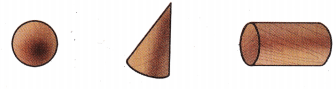A sphere has only a curved surface.
A ball is a toy that ¡s a sphere.A circle has only a curved surface.

HOME CONNECTION • Your child solved this problem by looking at models of three-dimensional solids and finding a model with only a curved surface.

Try Another Problem

Draw an object for the riddle. Use models of solids to help you.Question 1.
I am a block.
I have 6 faces that are squares.
Draw me.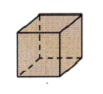Explanation:
A cube has 6 faces that are squares.

Question 2.
I am a hat.
I have I flat surface.
I have I curved surface.
Draw me.
ConeExplanation:
A cone has 1 flat surface and 1 curved surface.

Question 3.
I am a box.
I have 2 faces squares.
I have 4 faces rectangles.
Draw me.A cuboid has 2 faces squares and 4 faces rectangles.

Math Talk
Mathematical Processes

Making a model helps to solve a riddle to perform the best and make it as a easy way of understanding.

Share and Show

Draw an object for the riddle. Use models of solids to help you.

Question 4.
I am a can.
I have 2 flat surfaces.
I have I curved surface.
Draw me.
Cylinder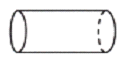A cylinder gas 2 flat surfaces and 1 curved surface.

Problem Solving

Question 5.
H.O.T.I am a box.
I have 2 faces that are triangles.
I have 3 faces that are rectangles.
Draw me.
Triangular PrismExplanation:
A triangular prism has 2 faces triangles and 3 faces rectangles.

Question 6.
H.O.T. Multi-Step
I am a block.
I have 6 faces.
I have 8 vertices.
Draw two different blocks I could be.
A cube has 6 faces and 8 vertices.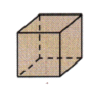Question 7.
Analyze Tim has a toy that is shaped like a sphere. Which toy does Tim have?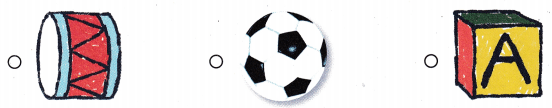A ball is a sphere shaped toy, Tim have a ball as a toy.

Question 8.
Mari picks a block with flat and curved surfaces. Which block does she pick?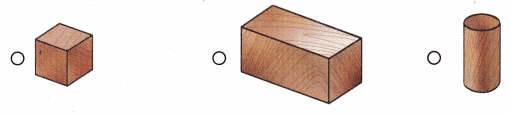A cylinder has both flat and curved surfaces, Mari picks cylinder.

Question 9.
Multi-Step David puts two blocks together to make a rectangular prism. Which blocks does David use?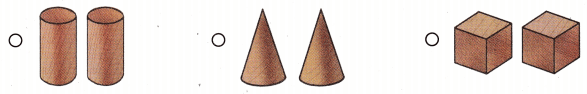To make a rectangular prism David need to use cubes.

Question 10.
Texas Test Prep Which solid is a triangular prism?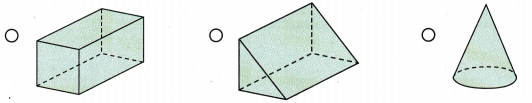A triangular prism is a 3D shape with two identical faces in the shape of a triangle joined with three triangles.

HOME CONNECTION • Have your child explain how he or she solved one of the problems in this lesson.

### Texas Go Math Grade 1 Lesson 15.4 Homework and Practice Answer Key

Draw an object for the riddle.

Question 1.
I have a curved surface.
I have no flat surfaces.
Draw me.
A sphere has 1 curved surface and no flat surface

Question 2.
I am a block.
I have I flat surface.
I have I curved surface.
Draw me.
ConeExplanation:
A cone has 1 flat surface and 1 curved surface.

Problem Solving

Question 3.
I am a block.
I have 3 faces that are rectangles.
I have 2 faces that are triangles.
Draw me.
Triangular PrismExplanation:
A triangular prism has 2 faces triangles and 3 faces rectangles.

Lesson Check

Question 4.
Wendy finds a toy that is shaped like a cube. Which toy does Wendy find?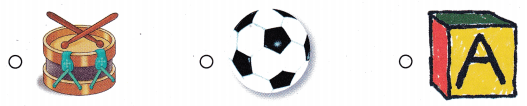Answer: A Block shaped like a cube

Question 5.
Multi-Step Winston puts four blocks together to make a tall cylinder. Which blocks does Winston use?